### Home > CALC > Chapter 3 > Lesson 3.3.2 > Problem3-104

3-104.
1. Define f(x) and g(x) so that h(x) = f(g(x)) for the following functions given that f(x) ≠ x and g(x) ≠ x. Homework Help ✎

1. h(x) = (2x − 5)3

2. h(x) = sin(3x − 1)

3.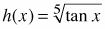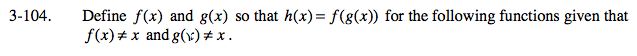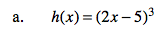g(x) = 2x − 5
f(x) = x³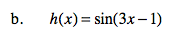g(x) is the inner function.
f(x) is the outer function.
The 'inner' function can usually be found 'inside' (parenthesis), |absolute value| or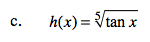Refer to previous hints.